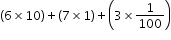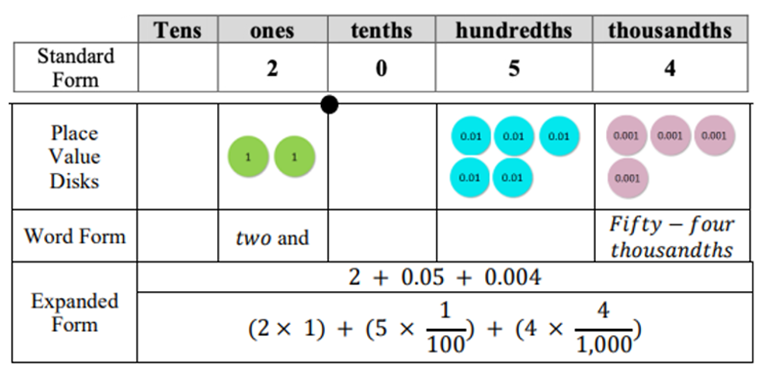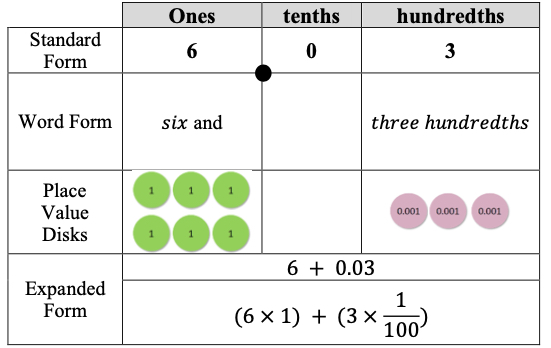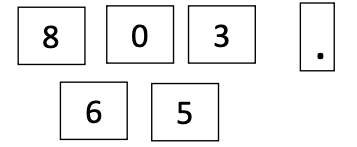# MA.5.NSO.1.2Export Print
Read and write multi-digit numbers with decimals to the thousandths using standard form, word form and expanded form.

### Examples

The number sixty-seven and three hundredths written in standard form is 67.03 and in expanded form is 60+7+0.03 or.
General Information
Subject Area: Mathematics (B.E.S.T.)
Strand: Number Sense and Operations
Status: State Board Approved

## Benchmark Instructional Guide

• NA

### Vertical Alignment

Previous Benchmarks

Next Benchmarks

### Purpose and Instructional Strategies

The purpose of this benchmark is for students to read numbers appropriately and to write numbers in all forms. Utilizing place value, students are expected to understand the value of tenths, hundredths, and thousandths, extending from their work to read and write whole numbers in any form in grade 4 (MA.4.NSO.1.2). Writing numbers in expanded form can help students see the relationship between decimals and fractions (MTR.5.1). Translating from written form to symbolic form builds the foundation for moving from written to algebraic form in grade 6 (MA.6.AR.1.1).
• Representing numbers in flexible ways will help students name, order, compare and operate with decimals (MTR.3.1).
• During instruction, teachers should relate all three forms using place value charts and base ten manipulatives (e.g., blocks) (MTR.3.1, MTR.4.1, MTR.5.1).

### Common Misconceptions or Errors

• Students may incorrectly read and write from expanded form if one of the digits is 0, like in the number 67.03 as used in the benchmark example. A common mistake that students make is to name the number as 67.3 because they do not see that 3 is the value of hundredths.

### Strategies to Support Tiered Instruction

• Instruction includes the use of place value understanding, models such as place value disks and decimal fractions to read and write multi-digit numbers with decimals to the thousandths using standard form, word form and expanded form when one of the digits in the decimal place values is 0.
• For example, write 2.054 in standard form, word form and expanded form using a place value chart.For example, the teacher uses decimal fractions and a place value chart to help students read 2.054, modeling how to write the decimal portion of the number as a fraction,
• $\frac{\text{54}}{\text{1,000}}$ and explaining that doing so helps us to read the decimal correctly. Also, the teacher explains that the word “and” is used for a portion of a number, decimal or fraction. Next, the teacher and students write 2.054 as 2 $\frac{\text{54}}{\text{1,000}}$ and read  the number as “two and fifty-four thousandths.”
• For example, write 6.03 in standard form, word form and expanded form using a place value chart.• For example, the teacher uses decimal fractions and a place value chart to help students read 6.03, while modeling how to write the decimal portion of the number as a fraction, $\frac{\text{3}}{\text{100}}$ and explaining that doing so helps us to read the decimal correctly. Also, the teacher explains that the word “and” is used for a portion of a number, decimal or fraction. Next, write 6.03 as 6 $\frac{\text{3}}{\text{100}}$ and read the number as “six and three hundredths.”

Use the number cards below to write a number in standard, word and expanded forms. You can use the cards in any order to make your number, but it must have a digit other than zero in the thousandths place.### Instructional Items

Instructional Item 1

Which shows the number below in word form?
(7 ×100)+(2×1)+(5× $\frac{\text{1}}{\text{10}}$)+(9× $\frac{\text{1}}{\text{1000}}$

• a. Seventy two and fifty nine thousands
• b. Seven hundred two and fiftynine hundredths
• c. Seven hundred two and five hundred nine thousandths
• d. Seventytwo and five hundred nine thousandths

Instructional Item 2

Write eight thousand and 2 hundredths in standard form.

*The strategies, tasks and items included in the B1G-M are examples and should not be considered comprehensive.

## Related Courses

This benchmark is part of these courses.
5012070: Grade Five Mathematics (Specifically in versions: 2014 - 2015, 2015 - 2022, 2022 and beyond (current))
7712060: Access Mathematics Grade 5 (Specifically in versions: 2014 - 2015, 2015 - 2018, 2018 - 2022, 2022 and beyond (current))
5012065: Grade 4 Accelerated Mathematics (Specifically in versions: 2019 - 2022, 2022 and beyond (current))
5012015: Foundational Skills in Mathematics 3-5 (Specifically in versions: 2019 - 2022, 2022 and beyond (current))

## Related Access Points

Alternate version of this benchmark for students with significant cognitive disabilities.
MA.5.NSO.1.AP.2: Read and generate multi-digit numbers with decimals to the hundredths using standard form and expanded form. Multi-digit numbers not to exceed 9.99.

## Related Resources

Vetted resources educators can use to teach the concepts and skills in this benchmark.

## Educational Game

Flower Power: An Ordering of Rational Numbers Game:

This is a fun and interactive game that helps students practice ordering rational numbers, including decimals, fractions, and percents. You are planting and harvesting flowers for cash. Allow the bee to pollinate, and you can multiply your crops and cash rewards!

Type: Educational Game

## Formative Assessments

Decimals in Expanded Form:

Students are asked to write numbers involving decimals in both standard form (as base ten numerals) and expanded form.

Type: Formative Assessment

Decimals in Number Name:

Students are asked to write the number name (word form) of a number when given the base-ten numeral (standard form).

Type: Formative Assessment

Students are read aloud four numbers and are asked to write the numbers using decimal notation. Then students are shown a number containing a decimal and asked to read it aloud.

Type: Formative Assessment

## Lesson Plans

Where's Your Place In the Kingdom of Decimals?:

This lesson has activities to review place value to the thousandths place. The teacher creates a kingdom of decimals in the classroom and opposing knights duel with their digits while reviewing the meaning of place value.

Type: Lesson Plan

Decimals Have a Point!:

This 5E lesson provides a framework for students to manipulate and understand the relationships between decimal place values through the thousandths. Students will have the opportunity to work with a concrete model that they will then use in a pictorial representation. This lesson is designed to be spread over 2, 45-minute sessions.

Type: Lesson Plan

Batting a Thousand(th):

This 5E lesson emphasizes the framework created in the lesson "Decimals Have a Point!" (ID: 30766) in which students learned to manipulate and understand the relationships between decimal place values through the thousandths. Students will have the opportunity to reference the pictorial model (see attached) to compare two decimals up to thousandths. This lesson is designed to be done in a 60 minute block.

Type: Lesson Plan

Secret Number-Place Value Game:

Students will be able to use decimals through the thousandths place to name numbers between whole numbers through the use of number generators and critically thinking about where to place numbers on a place value chart.

Type: Lesson Plan

Tacking on Decimals:

This lesson involves writing, reading and comparing decimals. It is a review of place value, and a Swat game at the end for skills practice.

Type: Lesson Plan

## Original Student Tutorial

Cracking the Decimal Code:

By the end of this tutorial, you will be able to read and write decimals to the thousandths using base-ten numerals, number names, and expanded form.

Type: Original Student Tutorial

## MFAS Formative Assessments

Decimals in Expanded Form:

Students are asked to write numbers involving decimals in both standard form (as base ten numerals) and expanded form.

Decimals in Number Name:

Students are asked to write the number name (word form) of a number when given the base-ten numeral (standard form).

Students are read aloud four numbers and are asked to write the numbers using decimal notation. Then students are shown a number containing a decimal and asked to read it aloud.

## Original Student Tutorials Mathematics - Grades K-5

Cracking the Decimal Code:

By the end of this tutorial, you will be able to read and write decimals to the thousandths using base-ten numerals, number names, and expanded form.

## Student Resources

Vetted resources students can use to learn the concepts and skills in this benchmark.

## Original Student Tutorial

Cracking the Decimal Code:

By the end of this tutorial, you will be able to read and write decimals to the thousandths using base-ten numerals, number names, and expanded form.

Type: Original Student Tutorial

## Educational Game

Flower Power: An Ordering of Rational Numbers Game:

This is a fun and interactive game that helps students practice ordering rational numbers, including decimals, fractions, and percents. You are planting and harvesting flowers for cash. Allow the bee to pollinate, and you can multiply your crops and cash rewards!

Type: Educational Game

## Parent Resources

Vetted resources caregivers can use to help students learn the concepts and skills in this benchmark.